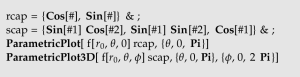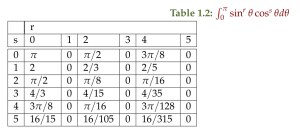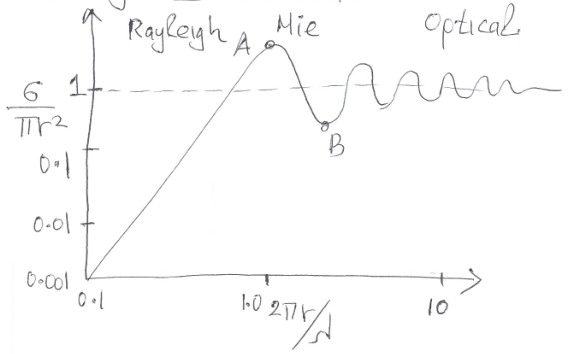## Updated notes for ece1229 antenna theoryI’ve now posted a first update of my notes for the antenna theory course that I am taking this term at UofT.

Unlike most of the other classes I have taken, I am not attempting to take comprehensive notes for this class. The class is taught on slides which go by faster than I can easily take notes for (and some of which match the textbook closely). In class I have annotated my copy of textbook with little details instead. This set of notes contains musings of details that were unclear, or in some cases, details that were provided in class, but are not in the text (and too long to pencil into my book), as well as some notes Geometric Algebra formalism for Maxwell’s equations with magnetic sources (something I’ve encountered for the first time in any real detail in this class).

The notes compilation linked above includes all of the following separate notes, some of which have been posted separately on this blog:

## Notes for ece1229 antenna theoryI’ve now posted a first set of notes for the antenna theory course that I am taking this term at UofT.

Unlike most of the other classes I have taken, I am not attempting to take comprehensive notes for this class. The class is taught on slides that match the textbook so closely, there is little value to me taking notes that just replicate the text. Instead, I am annotating my copy of textbook with little details instead. My usual notes collection for the class will contain musings of details that were unclear, or in some cases, details that were provided in class, but are not in the text (and too long to pencil into my book.)

• Reading notes for chapter 2 (Fundamental Parameters of Antennas) and chapter 3 (Radiation Integrals and Auxiliary Potential Functions) of the class text.
• Geometric Algebra musings.  How to do formulate Maxwell’s equations when magnetic sources are also included (those modeling magnetic dipoles).
• Some problems for chapter 2 content.

## Polarization review

It seems worthwhile to review how a generally polarized field phasor leads to linear, circular, and elliptic geometries.

The most general field polarized in the $$x, y$$ plane has the form

\begin{equation}\label{eqn:polarizationReview:20}
\BE
= \lr{ \xcap a e^{j \alpha} + \ycap b e^{j \beta} } e^{j \lr{ \omega t -k z }}
= \lr{ \xcap a e^{j \lr{\alpha – \beta}/2} + \ycap b e^{j \lr{ \beta – \alpha}/2} } e^{j \lr{ \omega t -k z + \lr{\alpha + \beta}/2 }}.
\end{equation}

Knowing to factor out the average phase angle above is only because I tried initially without that and things got ugly and messy. I guessed this would help (it does).

Let $$\boldsymbol{\mathcal{E}} = \text{Re} \BE = \xcap x + \ycap y$$, $$\theta = \omega t + (\alpha + \beta)/2$$, and $$\phi = (\alpha – \beta)/2$$, so that

\begin{equation}\label{eqn:polarizationReview:40}
\BE
= \lr{ \xcap a e^{j \phi} + \ycap b e^{-j \phi} } e^{j \theta }.
\end{equation}

The coordinates can now be read off

\begin{equation}\label{eqn:polarizationReview:60}
\frac{x}{a} = \cos\phi \cos\theta – \sin\phi \sin\theta
\end{equation}
\begin{equation}\label{eqn:polarizationReview:80}
\frac{y}{b} = \cos\phi \cos\theta + \sin\phi \sin\theta,
\end{equation}

or in matrix form

\begin{equation}\label{eqn:polarizationReview:100}
\begin{bmatrix}
x/a \\
y/b \\
\end{bmatrix}
=
\begin{bmatrix}
\cos\phi & – \sin\phi \\
\cos\phi & \sin\phi
\end{bmatrix}
\begin{bmatrix}
\cos\theta \\
\sin\theta
\end{bmatrix}.
\end{equation}

The goal is to eliminate all the $$\theta$$ (i.e. time dependence), converting the parametric relationship into a conic form.
Assuming that neither $$\cos\theta$$, nor $$\sin\theta$$ are zero for now (those are special cases and lead to linear polarization), inverting the matrix will allow the $$\theta$$ dependence to be eliminated

\begin{equation}\label{eqn:polarizationReview:120}
\inv{\sin\lr{ 2\phi }}
\begin{bmatrix}
\sin\phi & \sin\phi \\
– \cos\phi & \cos\phi
\end{bmatrix}
\begin{bmatrix}
x/a \\
y/b \\
\end{bmatrix}
=
\begin{bmatrix}
\cos\theta \\
\sin\theta
\end{bmatrix}.
\end{equation}

Squaring and summing both rows of these equation gives
\begin{equation}\label{eqn:polarizationReview:140}
\begin{aligned}
1
&=
\inv{\sin^2 \lr{ 2\phi}}
\lr{
\sin^2\phi
\lr{
\frac{x}{a}
+\frac{y}{b}
}^2
+
\cos^2\phi
\lr{
-\frac{x}{a}
+\frac{y}{b}
}^2
} \\
&=
\inv{\sin^2 \lr{ 2\phi}}
\lr{
\frac{x^2}{a^2}
+\frac{y^2}{b^2}
+2 \frac{x y}{a b} \lr{ \sin^2\phi – \cos^2\phi }
} \\
&=
\inv{\sin^2 \lr{ 2\phi}}
\lr{
\frac{x^2}{a^2}
+\frac{y^2}{b^2}
-2 \frac{x y}{a b} \cos \lr{2\phi}
}
\end{aligned}
\end{equation}

Time to summarize and handle the special cases.

1. To have $$\cos\phi = 0$$, the phase angles must satisfy $$\alpha – \beta = \lr{ 1 + 2 k } \pi, \, k \in \mathbb{Z}$$.

For this case \ref{eqn:polarizationReview:50} reduces to

\begin{equation}\label{eqn:polarizationReview:160}
-\frac{x}{a} = \frac{y}{b},
\end{equation}

which is just a line.

### Example.

Let $$\alpha = 0, \beta = -\pi$$, so that the phasor has the value

\begin{equation}\label{eqn:polarizationReview:260}
\BE = \lr{ \xcap a – \ycap b } e^{j \omega t}
\end{equation}

2. For have $$\sin\phi = 0$$, the phase angles must satisfy $$\alpha – \beta = 2 \pi k, \, k \in \mathbb{Z}$$.

For this case \ref{eqn:polarizationReview:60} and \ref{eqn:polarizationReview:80} reduce to

\begin{equation}\label{eqn:polarizationReview:180}
\frac{x}{a} = \frac{y}{b},
\end{equation}

also just a line.

### Example.

Let $$\alpha = \beta = 0$$, so that the phasor has the value

\begin{equation}\label{eqn:polarizationReview:280}
\BE = \lr{ \xcap a + \ycap b } e^{j \omega t}
\end{equation}

3. Last is the circular and elliptically polarized case. The system is clearly elliptically polarized if $$\cos(2 \phi) = 0$$, or $$\alpha – \beta = (\pi/2)( 1 + 2 k ), k \in \mathbb{Z}$$. When that is the case and $$a = b$$ also holds, the ellipse is a circle.

When the $$\cos( 2 \phi) = 0$$ condition does not hold, a rotation of coordinates

\begin{equation}\label{eqn:polarizationReview:200}
\begin{bmatrix}
x \\
y
\end{bmatrix}
=
\begin{bmatrix}
\cos\mu & \sin\mu \\
-\sin\mu & \cos\mu
\end{bmatrix}
\begin{bmatrix}
u \\
v
\end{bmatrix}
\end{equation}

where

\begin{equation}\label{eqn:polarizationReview:220}
\mu = \inv{2} \tan^{-1} \lr{ \frac{ 2 \cos (\alpha – \beta)}{b – a}}
\end{equation}

puts the trajectory into a standard (but messy) conic form

\begin{equation}\label{eqn:polarizationReview:240}
1 = \frac{u^2}{ab} \lr{
\frac{b}{a} \cos^2 \mu
+ \frac{a}{b} \sin^2 \mu
+ \inv{2} \sin\lr{2 \mu + \alpha – \beta}
}
+
\frac{v^2}{ab} \lr{
\frac{b}{a} \sin^2 \mu
+ \frac{a}{b} \cos^2 \mu
– \inv{2} \sin\lr{2 \mu + \alpha – \beta}
}
\end{equation}

It isn’t obvious to me that the factors of the $$u^2, v^2$$ terms are necessarily positive, which is required for the conic to be an ellipse and not a hyperbola.

### Circular polarization example.

With $$a = b = E_0$$, $$\alpha = 0$$, $$\beta = \pm \pi/2$$, all the circular polarization conditions are met, leaving the phasor with values

\begin{equation}\label{eqn:polarizationReview:300}
\BE = E_0 \lr{ \xcap \pm j \ycap } e^{j \omega t}
\end{equation}

## Fundamental parameters of antennas

This is my first set of notes for the UofT course ECE1229, Advanced Antenna Theory, taught by Prof. Eleftheriades, covering ch. 2  content.

Unlike most of the other classes I have taken, I am not attempting to take comprehensive notes for this class. The class is taught on slides that match the textbook so closely, there is little value to me taking notes that just replicate the text. Instead, I am annotating my copy of textbook with little details instead. My usual notes collection for the class will contain musings of details that were unclear, or in some cases, details that were provided in class, but are not in the text (and too long to pencil into my book.)

## Poynting vector

The Poynting vector was written in an unfamiliar form

\begin{equation}\label{eqn:chapter2Notes:560}
\boldsymbol{\mathcal{W}} = \boldsymbol{\mathcal{E}} \cross \boldsymbol{\mathcal{H}}.
\end{equation}

I can roll with the use of a different symbol (i.e. not $$\BS$$) for the Poynting vector, but I’m used to seeing a $$\frac{c}{4\pi}$$ factor ( and ). I remembered something like that in SI units too, so was slightly confused not to see it here.

Per  that something is a $$\mu_0$$, as in

\begin{equation}\label{eqn:chapter2Notes:580}
\boldsymbol{\mathcal{W}} = \inv{\mu_0} \boldsymbol{\mathcal{E}} \cross \boldsymbol{\mathcal{B}}.
\end{equation}

Note that the use of $$\boldsymbol{\mathcal{H}}$$ instead of $$\boldsymbol{\mathcal{B}}$$ is what wipes out the requirement for the $$\frac{1}{\mu_0}$$ term since $$\boldsymbol{\mathcal{H}} = \boldsymbol{\mathcal{B}}/\mu_0$$, assuming linear media, and no magnetization.

It was mentioned that

U(\theta, \phi)
=
\frac{r^2}{2 \eta_0} \Abs{ \BE( r, \theta, \phi) }^2
=
\frac{1}{2 \eta_0} \lr{ \Abs{ E_\theta(\theta, \phi) }^2 + \Abs{ E_\phi(\theta, \phi) }^2},
\end{equation}

where the intrinsic impedance of free space is

\eta_0 = \sqrt{\frac{\mu_0}{\epsilon_0}} = 377 \Omega.
\end{equation}

(this is also eq. 2-19 in the text.)

To get an understanding where this comes from, consider the far field radial solutions to the electric and magnetic dipole problems, which have the respective forms (from ) of

\begin{equation}\label{eqn:chapter2Notes:740}
\begin{aligned}
\boldsymbol{\mathcal{E}} &= -\frac{\mu_0 p_0 \omega^2 }{4 \pi } \frac{\sin\theta}{r} \cos\lr{w t – k r} \thetacap \\
\boldsymbol{\mathcal{B}} &= -\frac{\mu_0 p_0 \omega^2 }{4 \pi c} \frac{\sin\theta}{r} \cos\lr{w t – k r} \phicap \\
\end{aligned}
\end{equation}
\begin{equation}\label{eqn:chapter2Notes:760}
\begin{aligned}
\boldsymbol{\mathcal{E}} &= \frac{\mu_0 m_0 \omega^2 }{4 \pi c} \frac{\sin\theta}{r} \cos\lr{w t – k r} \phicap \\
\boldsymbol{\mathcal{B}} &= -\frac{\mu_0 m_0 \omega^2 }{4 \pi c^2} \frac{\sin\theta}{r} \cos\lr{w t – k r} \thetacap \\
\end{aligned}
\end{equation}

In neither case is there a component in the direction of propagation, and in both cases (using $$\mu_0 \epsilon_0 = 1/c^2$$)

\begin{equation}\label{eqn:chapter2Notes:780}
\Abs{\boldsymbol{\mathcal{H}}}
= \frac{\Abs{\boldsymbol{\mathcal{E}}}}{\mu_0 c}
= \Abs{\boldsymbol{\mathcal{E}}} \sqrt{\frac{\epsilon_0}{\mu_0}}
= \inv{\eta_0}\Abs{\boldsymbol{\mathcal{E}}} .
\end{equation}

A superposition of the phasors for such dipole fields, in the far field, will have the form

\begin{equation}\label{eqn:chapter2Notes:800}
\begin{aligned}
\BE &= \inv{r} \lr{ E_\theta(\theta, \phi) \thetacap + E_\phi(\theta, \phi) \phicap } \\
\BB &= \inv{r c} \lr{ E_\theta(\theta, \phi) \thetacap – E_\phi(\theta, \phi) \phicap },
\end{aligned}
\end{equation}

with a corresponding time averaged Poynting vector

\begin{equation}\label{eqn:chapter2Notes:820}
\begin{aligned}
\BW_{\textrm{av}}
&= \inv{2 \mu_0} \BE \cross \BB^\conj \\
&=
\inv{2 \mu_0 c r^2}
\lr{ E_\theta \thetacap + E_\phi \phicap } \cross
\lr{ E_\theta^\conj \thetacap – E_\phi^\conj \phicap } \\
&=
\frac{\thetacap \cross \phicap}{2 \mu_0 c r^2}
\lr{ \Abs{E_\theta}^2 + \Abs{E_\phi}^2 } \\
&=
\frac{\rcap}{2 \eta_0 r^2}
\lr{ \Abs{E_\theta}^2 + \Abs{E_\phi}^2 },
\end{aligned}
\end{equation}

verifying \ref{eqn:advancedantennaL1:20} for a superposition of electric and magnetic dipole fields. This can likely be shown for more general fields too.

## Field plots

We can plot the fields, or intensity (or log plots in dB of these).
It is pointed out in  that when there is $$r$$ dependence these plots are done by considering the values of at fixed $$r$$.

The field plots are conceptually the simplest, since that vector parameterizes
a surface. Any such radial field with magnitude $$f(r, \theta, \phi)$$ can
be plotted in Mathematica in the $$\phi = 0$$ plane at $$r = r_0$$, or in
3D (respectively, but also at $$r = r_0$$) with code like that of the
following listingIntensity plots can use the same code, with the only difference being the interpretation. The surface doesn’t represent the value of a vector valued radial function, but is the magnitude of a scalar valued function evaluated at $$f( r_0, \theta, \phi)$$.

The surfaces for $$U = \sin\theta, \sin^2\theta$$ in the plane are parametrically plotted in fig. 2, and for cosines in fig. 1 to compare with textbook figures.

Visualizations of $$U = \sin^2 \theta$$ and $$U = \cos^2 \theta$$ can be found in fig. 3 and fig. 4 respectively. Even for such simple functions these look pretty cool.

## dB vs dBi

Note that dBi is used to indicate that the gain is with respect to an “isotropic” radiator.
This is detailed more in .

## Trig integrals

Tables 1.1 and 1.2 produced with tableOfTrigIntegrals.nb have some of the sine and cosine integrals that are pervasive in this chapter.## Polarization vectors

The text introduces polarization vectors $$\rhocap$$ , but doesn’t spell out their form. Consider a plane wave field of the form

\begin{equation}\label{eqn:chapter2Notes:840}
\BE
=
E_x e^{j \phi_x} e^{j \lr{ \omega t – k z }} \xcap
+
E_y e^{j \phi_y} e^{j \lr{ \omega t – k z }} \ycap.
\end{equation}

The $$x, y$$ plane directionality of this phasor can be written

\begin{equation}\label{eqn:chapter2Notes:860}
\Brho =
E_x e^{j \phi_x} \xcap
+
E_y e^{j \phi_y} \ycap,
\end{equation}

so that

\begin{equation}\label{eqn:chapter2Notes:880}
\BE = \Brho e^{j \lr{ \omega t – k z }}.
\end{equation}

Separating this direction and magnitude into factors

\begin{equation}\label{eqn:chapter2Notes:900}
\Brho = \Abs{\BE} \rhocap,
\end{equation}

allows the phasor to be expressed as

\begin{equation}\label{eqn:chapter2Notes:920}
\BE = \rhocap \Abs{\BE} e^{j \lr{ \omega t – k z }}.
\end{equation}

As an example, suppose that $$E_x = E_y$$, and set $$\phi_x = 0$$. Then

\begin{equation}\label{eqn:chapter2Notes:940}
\rhocap = \xcap + \ycap e^{j \phi_y}.
\end{equation}

## Phasor power

In section 2.13 the phasor power is written as

\begin{equation}\label{eqn:chapter2Notes:620}
I^2 R/2,
\end{equation}

where $$I, R$$ are the magnitudes of phasors in the circuit.

I vaguely recall this relation, but had to refer back to  for the details.
This relation expresses average power over a period associated with the frequency of the phasor

\begin{equation}\label{eqn:chapter2Notes:640}
\begin{aligned}
P
&= \inv{T} \int_{t_0}^{t_0 + T} p(t) dt \\
&= \inv{T} \int_{t_0}^{t_0 + T} \Abs{\BV} \cos\lr{ \omega t + \phi_V }
\Abs{\BI} \cos\lr{ \omega t + \phi_I} dt \\
&= \inv{T} \int_{t_0}^{t_0 + T} \Abs{\BV} \Abs{\BI}
\lr{
\cos\lr{ \phi_V – \phi_I } + \cos\lr{ 2 \omega t + \phi_V + \phi_I}
}
dt \\
&= \inv{2} \Abs{\BV} \Abs{\BI} \cos\lr{ \phi_V – \phi_I }.
\end{aligned}
\end{equation}

Introducing the impedance for this circuit element

\begin{equation}\label{eqn:chapter2Notes:660}
\BZ = \frac{ \Abs{\BV} e^{j\phi_V} }{ \Abs{\BI} e^{j\phi_I} } = \frac{\Abs{\BV}}{\Abs{\BI}} e^{j\lr{\phi_V – \phi_I}},
\end{equation}

this average power can be written in phasor form

\begin{equation}\label{eqn:chapter2Notes:680}
\BP = \inv{2} \Abs{\BI}^2 \BZ,
\end{equation}

with
\begin{equation}\label{eqn:chapter2Notes:700}
P = \textrm{Re} \BP.
\end{equation}

Observe that we have to be careful to use the absolute value of the current phasor $$\BI$$, since $$\BI^2$$ differs in phase from $$\Abs{\BI}^2$$. This explains the conjugation in the  definition of complex power, which had the form

\begin{equation}\label{eqn:chapter2Notes:720}
\BS = \BV_{\textrm{rms}} \BI^\conj_{\textrm{rms}}.
\end{equation}

### Flat plate.

\begin{equation}\label{eqn:chapter2Notes:960}
\sigma_{\textrm{max}} = \frac{4 \pi \lr{L W}^2}{\lambda^2}
\end{equation}

### Sphere.

In the optical limit the radar cross section for a sphere

\begin{equation}\label{eqn:chapter2Notes:980}
\sigma_{\textrm{max}} = \pi r^2
\end{equation}

Note that this is smaller than the physical area $$4 \pi r^2$$.

### Cylinder.

\begin{equation}\label{eqn:chapter2Notes:1000}
\sigma_{\textrm{max}} = \frac{ 2 \pi r h^2}{\lambda}
\end{equation}

### Tridedral corner reflector

\begin{equation}\label{eqn:chapter2Notes:1020}
\sigma_{\textrm{max}} = \frac{ 4 \pi L^4}{3 \lambda^2}
\end{equation}

## Scattering from a sphere vs frequency

Frequency dependence of spherical scattering is sketched in fig. 10.

• Low frequency (or small particles): Rayleigh\begin{equation}\label{eqn:chapter2Notes:1040}
\sigma = \lr{\pi r^2} 7.11 \lr{\kappa r}^4, \qquad \kappa = 2 \pi/\lambda.
\end{equation}
• Mie scattering (resonance),\begin{equation}\label{eqn:chapter2Notes:1060}
\sigma_{\textrm{max}}(A) = 4 \pi r^2
\end{equation}
\begin{equation}\label{eqn:chapter2Notes:1080}
\sigma_{\textrm{max}}(B) = 0.26 \pi r^2.
\end{equation}
• optical limit ( $$r \gg \lambda$$ )\begin{equation}\label{eqn:chapter2Notes:1100}
\sigma = \pi r^2.
\end{equation}fig 10. Scattering from a sphere vs frequency (from Prof. Eleftheriades’ class notes).

FIXME: Do I have a derivation of this in my optics notes?

## Notation

• Time average.
and the text  use square brackets $$[\cdots]$$ for time averages, not $$<\cdots>$$. Was that an engineering convention?
writes $$\Omega$$ as a circle floating above a face up square bracket, as in fig. 1, and $$\sigma$$ like a number 6, as in fig. 1.
• Bold vectors are usually phasors, with (bold) calligraphic script used for the time domain fields. Example: $$\BE(x,y,z,t) = \ecap E(x,y) e^{j \lr{\omega t – k z}}, \boldsymbol{\mathcal{E}}(x, y, z, t) = \textrm{Re} \BE$$.

# References

 Constantine A Balanis. Antenna theory: analysis and design. John Wiley \& Sons, 3rd edition, 2005.

 David Jeffrey Griffiths and Reed College. Introduction to electrodynamics. Prentice hall Upper Saddle River, NJ, 3rd edition, 1999.

 J.D. Irwin. Basic Engineering Circuit Analysis. MacMillian, 1993.

 JD Jackson. Classical Electrodynamics. John Wiley and Sons, 2nd edition, 1975.

 L.D. Landau and E.M. Lifshitz. The classical theory of fields. Butterworth-Heinemann, 1980. ISBN 0750627689.